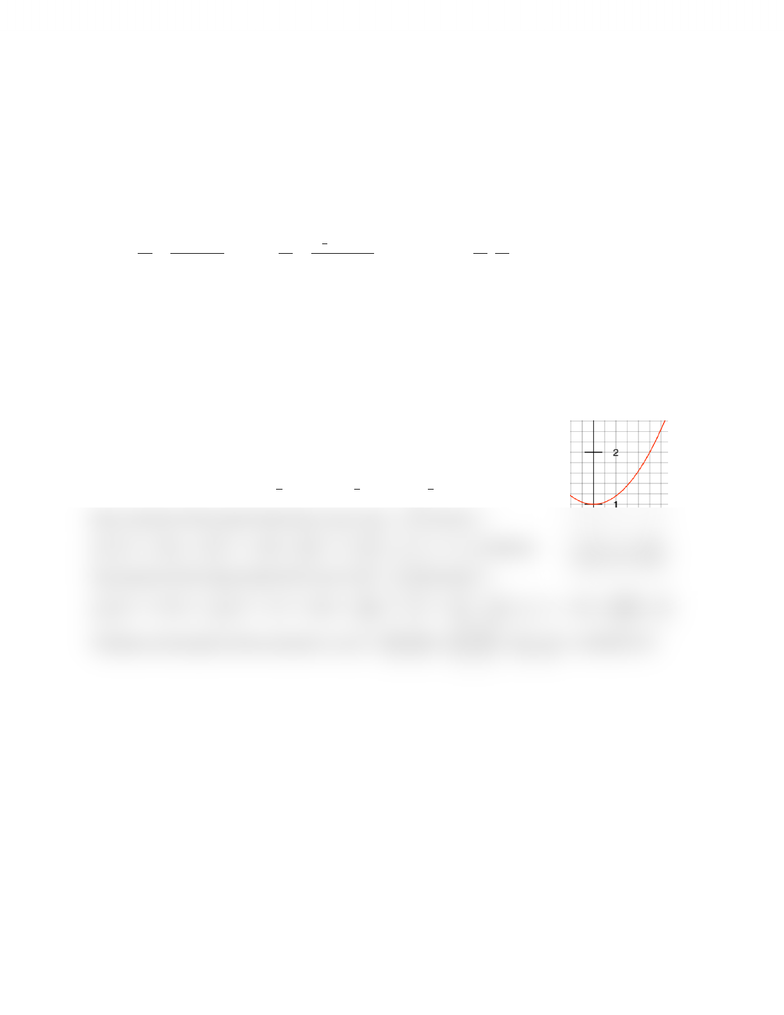Class Notes (1,100,000)
CA (630,000)
UTSG (50,000)
MAT (4,000)
MAT136H1 (900)
all (200)
Lecture

MAT136H1 Lecture Notes - Centroid

Department
Mathematics
Course Code
MAT136H1
Professor
all

This preview shows half of the first page. to view the full 1 pages of the document.8.3 Challenging Integral Applications
Physics & Engineering Applications
Question #6 (Medium): Finding the Coordinate of the Centroid
Strategy
For a region bound by continuous functions, the location for the coordinate for the centroid is calculated
as:  


and


. Thus,
Sample Question
Sketch the area bound by the function and visually estimate the location of the centroid. Then
determine the exact coordinate the centroid.
, , ,
Solution
From the graph, the bound region is right and down heavy. So it is   and
and can be guess to be around   and 
The total mass is calculated as the whole area of the bound region. Here,


Now, moment of the system about the x-axis is: 


. Likewise,
the moment of the system about the y-axis is:







Therefore, the location of the centroid is:




You're Reading a Preview

Unlock to view full version